## Sin, Cos and Tan

Sin, Cos and Tan A-Level Maths,

On a set of axes, angles are measured anti-clockwise from the positive x-axis. So 30° would be drawn as follows: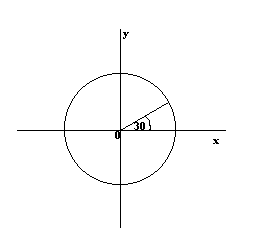The angles which lie between 0° and 90° are said to lie in the first quadrant. The angles between 90° and 180° are in the second quadrant, angles between 180° and 270° are in the third quadrant and angles between 270° and 360° are in the fourth quadrant: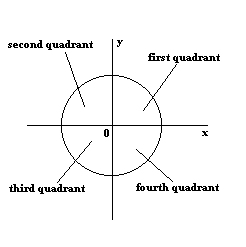In the first quadrant, the values for sin, cos and tan are positive.
In the second quadrant, the values for sin are positive only.
In the third quadrant, the values for tan are positive only.
In the fourth quadrant, the values for cos are positive only.

This can be summed up as follows: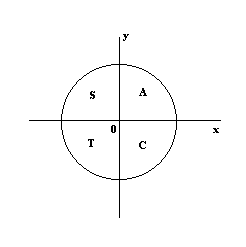In the fourth quadrant, Cos is positive, in the first, All are positive, in the second, Sin is positive and in the third quadrant, Tan is positive. This is easy to remember, since it spells "cast".

Related Angles

The sines, cosines and tangents of some angles are equal to the sines, cosines and tangents of other angles. For example, cos(-30°) = cos(30°) and cos(30°) = cos(390°) . In the following diagrams, the sines, cosines and tangents of each of the shaded angles have the same magnitude (the same angle in each diagram):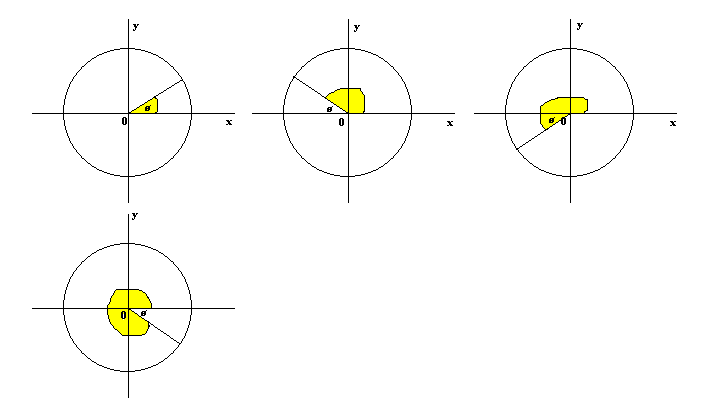For example, if q= 30°,
sin30° = 0.5
sin150° = 0.5
sin210° = -0.5
sin330° = -0.5

These angles are "related angles" and their cosines and tangents will be related in a similar way. Note that the signs of the sines (/cosines/tangents) are found using the "cast" rule.

Solving Equations

#### Example

Solve the equation sinq = 0.6428, for 0 < q < 360°

Take "arcsin" of both sides: q = arcsin(0.6428)
= 40°, 140°, 400°, ...
but the question asks for solutions between 0 and 360°, so the answer is 40° and 140° .

Graphing sin, cos and tan

The following are graphs of sin, cos & tan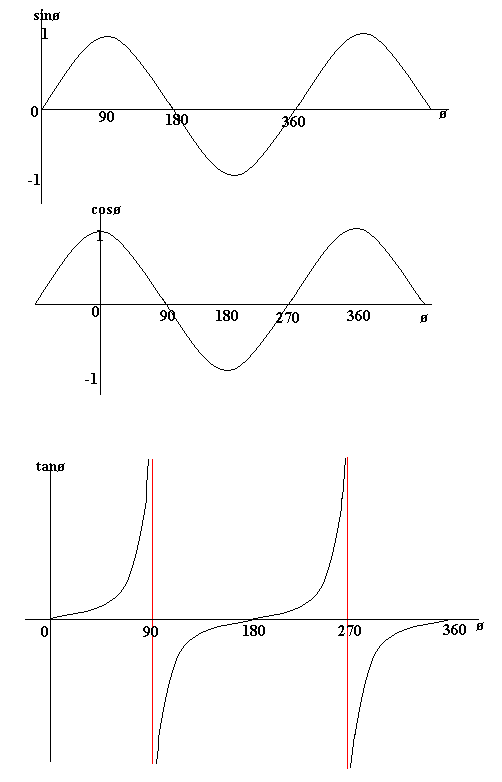Points to note

The graphs of sin and cos are periodic, with period of 360° (in other words the graphs repeat themselves every 360°).
The graph of cos the same as the graph of sin though it is shifted 90° to the right/ left. For this reason sinx = cos(90 - x) and cosx = sin(90 - x)

Note that cos is an even function:- it is symmetrical in the y-axis. sin is an odd function.
The graph of tan has asymptotes. An asymptote is a line which the graph gets very close to, but does not touch. The red lines are asymptotes.

These graphs obey the usual laws of graph transformations.

Arcsin, arccos, arctan

Arcsin is another way of writing the inverse of sin, arccos means the inverse of cos and arctan means the inverse of tan. For example, arcsin(0.5) = 30° . However, although this is true, we also know that sin(150°) = 0.5 (using the idea of related angles and the "cast rule"). If we continue moving round the "unit circle" (the circle with radius 1 that we have been drawing angles on above), then we find that sin(390°) is also 0.5 .
So we can write arcsin(0.5) = 30°, 150°, 390°, ...

It is possible to draw graphs of arcsin, arccos and arctan and you may need to know how to do this.